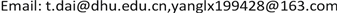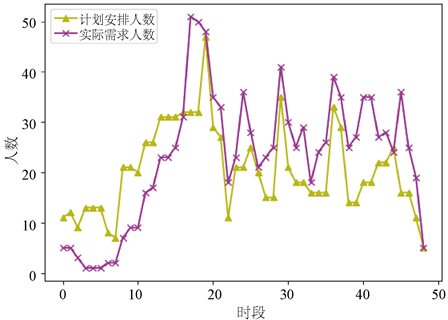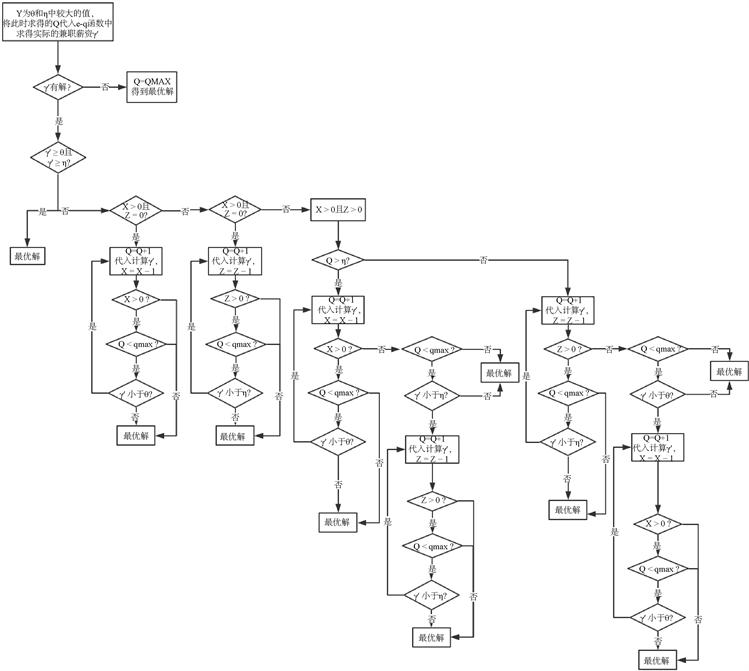﻿ 考虑兼职的客服排班实时调整研究 Study on Real-Time Adjustment of Customer Service Scheduling Considering Part-Time Staff

Service Science and Management
Vol. 09  No. 01 ( 2020 ), Article ID: 33659 , 9 pages
10.12677/SSEM.2020.91003

Study on Real-Time Adjustment of Customer Service Scheduling Considering Part-Time Staff

Tao Dai, Liuxiao YangReceived: Dec. 9th, 2019; accepted: Dec. 20th, 2019; published: Dec. 27th, 2019ABSTRACT

With the development of technology, the application of call centers is becoming more and more widespread. At the same time as the scale of enterprises is gradually expanding, the scheduling of customer service has also ushered in new challenges. In the daily operation of the customer service center, the amount of customer consultation will fluctuate on a large scale, resulting in an imbalance between the number of originally planned customer service and the actual manpower requirements, which will cause problems such as excessive customer waiting time or redundant staffing. Aiming at this problem, combined with the current development trend of the part-time market size, a real-time adjustment model considering part-time employment is established with the goal of minimizing costs, and the impact of part-time market structure and part-time job sensitivity on salary is considered. The calculation example shows that the real-time scheduling adjustment model can obtain the scheduling adjustment plan that meets the service level requirements at a lower cost.

Keywords:Part-Time Staff, Customer Service Scheduling, Real-Time Adjustment, Cost Minimization1. 引言

2. 考虑兼职的客服排班实时调整模型

2.1. 问题背景Figure 1. Relationship between hourly pay and number of part-time candidates

$q=\left\{\begin{array}{l}0,e\in \left[0,EMIN\right)\\ k\left(e-EMIN\right),e\in \left[EMIN,EMAX\right)\\ k\left(EMAX-EMIN\right),e\in \left[EMAX,\infty \right)\end{array}$ (2.1)

2.2. 实时调整模型建立

2.2.1. 模型假设与符号定义

(1) 任何班次的客服加班时长不超过8个时段(0.5小时为1个时段)；

(2) 其它班组的支援人员，统一的工作时间是16个时段；

(3) 在人力冗余时，若某班次部分客服提前下班时的上班时间不足16个时段，则需在人力短缺时继续上班满16个时段，但不再加班；

(4) 客服加班不超过8个时段，且必须工作16个时段才有机会提前下班；

(5) 对于已确定提前下班客服，当天不再回去工作；

(6) 客户在遇到呼叫占线的情况时等待达到其平均愿意等待时间后即放弃；

(7) 客服平均服务时间比客户平均愿意等待的时间短。

${X}_{it}=\left\{\begin{array}{l}{m}_{i}-{Z}_{i,\lambda -1}-\underset{k=1}{\overset{t-{L}_{i}-1}{\sum }}{y}_{ik},\forall i<{M}_{0},t\ge \mathrm{max}\left\{\lambda ,{L}_{i}+1\right\}\\ {m}_{i}-\underset{k=1}{\overset{t-{L}_{i}-1}{\sum }}{y}_{ik},\forall i>{M}_{0},t\ge {L}_{i}+1\\ 0,\forall i,t\le {L}_{i}\end{array}$ (2.3)

2.2.2. 模型建立

$\begin{array}{l}\mathrm{min}\underset{i=1}{\overset{{M}_{0}}{\sum }}\underset{t=2}{\overset{\lambda -1}{\sum }}{p}_{it}\left({Z}_{it}-{Z}_{i,t-1}\right)-\underset{i=1}{\overset{{M}_{0}}{\sum }}\underset{t={S}_{i}+16}{\overset{{L}_{i}}{\sum }}{s}_{i}\left({Z}_{it}-{Z}_{i,{S}_{i}+15}\right)\\ \text{\hspace{0.17em}}+\underset{i=1}{\overset{M}{\sum }}\underset{\lambda }{\overset{T-1}{\sum }}{p}_{it}\left({X}_{it}-{X}_{i,t+1}\right)+\underset{i=1}{\overset{M}{\sum }}\underset{\lambda }{\overset{T}{\sum }}{o}_{i}{X}_{it}+\underset{t=1}{\overset{T}{\sum }}\underset{c=1}{\overset{2}{\sum }}{e}_{tc}{Q}_{tc}\end{array}$ (2.4)

${Z}_{i,t+1}\ge {Z}_{it},\forall i<{M}_{0},{S}_{i}\le t<\mathrm{min}\left\{\lambda ,{L}_{i}\right\}$ (2.5)

${Z}_{it}={Z}_{i,t-1,}\forall i<{M}_{0},\mathrm{min}\left\{\lambda ,{L}_{i}\right\}\le t\le \mathrm{max}\left\{\lambda ,{L}_{i}\right\}$ (2.6)

${Z}_{it}\le {m}_{i},\forall i<{M}_{0},t=\mathrm{min}\left\{\lambda -1,{L}_{i}\right\}$ (2.7)

${P}_{t}=\sum {Z}_{i,{S}_{i}+15},\lambda \le t\le \lambda +15,{S}_{i}+15\le \lambda$ (2.8)

${X}_{it}\ge {X}_{i,t+1},\forall i,t>{L}_{i}$ (2.9)

${X}_{it}=0,\forall i,t<{L}_{i}+1$ (2.10)

${X}_{it}\le {m}_{i}-{Z}_{i,\lambda -1},\forall i<{M}_{0},t={L}_{i}+1$ (2.11)

${X}_{it}\le {m}_{i},\forall i>{M}_{0},t={L}_{i}+1$ (2.12)

$\underset{i=1}{\overset{{M}_{0}}{\sum }}{Z}_{it}\le \mathrm{max}\left\{-\alpha {\delta }_{t},0\right\},\forall t\le \lambda$ (2.13)

${P}_{t}+\underset{i=1}{\overset{M}{\sum }}\underset{\lambda }{\overset{T}{\sum }}{X}_{it}+\underset{\mathrm{max}\left\{0,t-15\right\}}{\overset{t}{\sum }}\underset{c=1}{\overset{\text{2}}{\sum }}{Q}_{tc}\ge \alpha {\delta }_{t},\forall t$ (2.14)

${Q}_{tc}\le QMA{X}_{tc},\forall t,\forall c$ (2.15)

${P}_{t},{X}_{it},{Z}_{it},{Q}_{tc},QMA{X}_{tc},EMI{N}_{c},EMA{X}_{c}\in {Z}^{+}\cup \left\{0\right\},\forall i,t$ (2.16)

3. 模型应用与求解Figure 2. Comparison of actual demand quantity and planned arrangement quantityFigure 3. Solution steps for optimal solution of mixed model

$k=1$$EMAX=16$$EMIN=10$，从而可得 $QMAX=6$

$q=\left\{\begin{array}{l}0,e\in \left[0,\text{10}\right)\\ e-\text{10},e\in \left[\text{10},\text{16}\right)\\ \text{6},e\in \left[\text{16},\infty \right)\end{array}$ (3.1)

(1.1) $X=2,Q=5,{\gamma }^{\prime }=15$

(1.2) $X=1,Q=6,{\gamma }^{\prime }=16$

(1.3) $X=0,Q=7,{\gamma }^{\prime }=17$

(2.1) $Z=4,Q=8,{\gamma }^{\prime }=18$

(2.2) $Z=3,Q=9,{\gamma }^{\prime }=19$

(2.3) $Z=2,Q=10,{\gamma }^{\prime }=20$

$X=0;Z=2,Q=10,{\gamma }^{\prime }=20$ 该时段的调整成本为240元。Figure 4. Comparison of the number of adjusted customer service staff

4. 总结与展望

Study on Real-Time Adjustment of Customer Service Scheduling Considering Part-Time Staff[J]. 服务科学和管理, 2020, 09(01): 26-34. https://doi.org/10.12677/SSEM.2020.91003

1. 1. 孙伟, 苏浩. 浅谈客户服务在企业发展中的作用[J]. 财经界(学术版), 2011(18): 109-109.

2. 2. 沈颂东, 丛丽. 呼叫中心服务质量测评模型的构建与分析[J]. 经济管理, 2011, 33(11): 54-60.

3. 3. Mehrotra, V., Wright, C. and Patel, S. (2009) An Investigation into the Business Processes, Job Descriptions, and Human Resource Factors Associated with Successful Workforce Management Practice. Working Paper, San Francisco State University, San Francisco, CA.

4. 4. 阚泽正, 金兆正, 杨紫淇, 赵礼强. 零工经济背景下大学生兼职市场调研分析[J]. 现代商业, 2018(23): 164-165.

5. 5. Warburg, V., Hansen, T.G., Larsen, A., Norman, H. and Andersson, E. (2008) Dynamic Airline Sche-duling: An Analysis of the Potentials of Refleeting and Retiming. Journal of Air Transport Management, 14, 163-167.
https://doi.org/10.1016/j.jairtraman.2008.03.004

6. 6. Easton, F.F. and Goodale, J.C. (2005) Schedule Recovery: Unplanned Absences in Service Operations. Decision Sciences, 36, 459-488.
https://doi.org/10.1111/j.1540-5414.2005.00080.x

7. 7. Gans, N., Shen, H. and Zhou, Y.P. (2015) Parametric Stochastic Programming Models for Call Center Workforce Scheduling. Manufacturing & Service Operations Man-agement, 17, 1-33.
https://doi.org/10.1287/msom.2015.0546

8. 8. Liao, S.Q. (2011) Staffing and Shift-Scheduling of Call Centers under Call Arrival Rate Uncertainty. Ecole Centrale Paris, Châtenay-Malabry.

9. 9. Mehrotra, V., Ozluk, O. and Saltzman, R. (2010) Intelligent Procedures for Intra-Day Updating of Call Center Agent Schedules. Production and Operations Management, 19, 353-367.
https://doi.org/10.1111/j.1937-5956.2009.01097.x